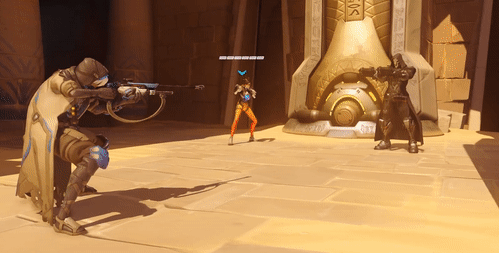•• Merch
• Donate
• SAT Prep

IntroductionIntroduction# Absolute Value

INTRO
Absolute value ignores a number’s direction (+/-) from 0 and just tells us how far the number is from 0.
It's kind of like how a video game character's overall power ignores the type of impact (❤️/🗡️) and is just focused on how much impact they have relative to 0.
Attackers have a negative effect on health, and healers have a positive effect on health.### Attacking 🗡️### Healing ❤️

But when we compare overall power, we just look at how much they change health by. Click/tap on some of the characters below to see how powerful they are!### Is Absolute Value Always Positive?

Since , and zero is neither negative or positive, the absolute value of any number is always either positive or zero.
Check out our
Calculator
or explore our
Lesson
and
Practice

CALCULATOR

## Absolute Value Calculator

Calculate the absolute value of:
KEY STEPS

## How to Calculate Absolute Value

### Step 1. Evaluate the expression inside the bars.

If there's only one number, move on to Step 2.

### Step 2. Drop the negative sign.

Absolute value is like the overall power of a number. It ignores the direction from 0 and just tells us how far it is from 0.
LESSON
Absolute Value

## Absolute Value

Just like how a character’s overall power ignores the type of impact (❤️/🗡️) and just describes how much impact they have relative to 0, absolute value ignores a number’s direction (+/-) from 0 and just tells us how far it is from 0.

We represent the absolute value of a number or expression by surrounding it with two vertical lines.
 Expression Meaning The absolute value of 5 is 5. The absolute value of -5 is 5. The negative of the absolute value of 5 is -5. The negative of the absolute value of -5 is -5.
Let’s walk through some absolute value example problems!
PRACTICE
Absolute Value

## Practice: Calculating Absolute Value

Question 1 of 11:

### Step 1. Evaluate the expression inside the bars.

Since there's only one number between the bars, we just move on to Step 2.

CONCLUSION
Incredible job, look at you go! Thanks for checking out this lesson ☺️🙏. Where to next?Leave Feedback